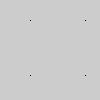Name

### point()

Examples```noSmooth()
point(30, 20)
point(85, 20)
point(85, 75)
point(30, 75)
``````size(100, 100, P3D)
noSmooth()
point(30, 20, -50)
point(85, 20, -50)
point(85, 75, -50)
point(30, 75, -50)
```
Description Draws a point, a coordinate in space at the dimension of one pixel. The first parameter is the horizontal value for the point, the second value is the vertical value for the point, and the optional third value is the depth value. Drawing this shape in 3D with the z parameter requires the P3D parameter in combination with size() as shown in the above example.
Syntax
```point(x, y)
point(x, y, z)
```
Parameters
x float: x-coordinate of the point float: y-coordinate of the point float: z-coordinate of the point

Updated on Mon Sep 21 15:53:25 2020.

If you see any errors or have comments, please let us know.